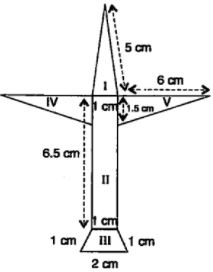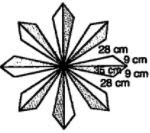# NCERT Solutions for Chapter 12 Heron's Formula Class 9 Maths

 Chapter Name NCERT Solutions for Chapter 12 Heron's Formula Class Class 9 Topics Covered Heron's Formula for different shapes Related Study Materials NCERT Solutions for Class 9 MathsNCERT Solutions for Class 9Revision Notes for Chapter 12 Heron's Formula Class 9 MathsImportant Questions for Chapter 12 Heron's Formula Class 9 MathsMCQ for for Chapter 12 Heron's Formula Class 9 Maths

## Short Revision for Ch 12 Heron's Formula Class 9 Maths

1. Area of a triangle = 1/2 × base×height.
2. Heron derived a formula to obtain the area of a triangle.
3. If the sides of a triangle are a, b and c, then Heron's formula to calculate the area of the triangle is given below :4. 's' represents in Heron's formula the semi-perimeter of the triangle.
5. Area of a quadrilateral whose all the sides and one diagonal are given, can be calculated by dividing it into triangles and then using Heron's formula.
6. Area of a scalene triangle can be calculated easily by Heron's formula.

### Exercise: 12.1

1. A traffic signal board, indicating ‘SCHOOL AHEAD’, is an equilateral triangle with side ‘a’. Find the area of the signal board, using Heron’s formula. If its perimeter is 180 cm, what will be the area of the signal board?

2. The triangular side walls of a flyover have been used for advertisements. The sides of the walls are 122 m, 22 m and 120 m (see Fig. 12.9). The advertisements yield an earning of ₹5000 per m2 per year. A company hired one of its walls for 3 months. How much rent did it pay?

3. There is a slide in a park. One of its side walls has been painted in some colour with a message “KEEP THE PARK GREEN AND CLEAN” (see Fig. 12.10 ). If the sides of the wall are 15 m, 11 m and 6 m, find the area painted in colour.

4. Find the area of a triangle two sides of which are 18 cm and 10 cm and the perimeter is 42cm.

5. Sides of a triangle are in the ratio of 12 : 17 : 25 and its perimeter is 540cm. Find its area.

6. An isosceles triangle has perimeter 30 cm and each of the equal sides is 12 cm. Find the area of the triangle.

### Exercise 12.2

1. A park, in the shape of a quadrilateral ABCD, has C = 90°, AB = 9 m, BC = 12 m, CD = 5 m and AD = 8 m. How much area does it occupy?

2. Find the area of a quadrilateral ABCD in which AB = 3 cm, BC = 4 cm, CD = 4 cm, DA = 5 cm and AC = 5 cm.

3. Radha made a picture of an aeroplane with coloured paper as shown in Fig 12.15. Find the total area of the paper used.4. A triangle and a parallelogram have the same base and the same area. If the sides of the triangle are 26 cm, 28 cm and 30 cm, and the parallelogram stands on the base 28 cm, find the height of the parallelogram.

5. A rhombus shaped field has green grass for 18 cows to graze. If each side of the rhombus is 30 m and its longer diagonal is 48 m, how much area of grass field will each cow be getting?

6. An umbrella is made by stitching 10 triangular pieces of cloth of two different colours (see Fig.12.16), each piece measuring 20 cm, 50 cm and 50 cm. How much cloth of each colour is required for the umbrella?

7. A kite in the shape of a square with a diagonal 32 cm and an isosceles triangle of base 8 cm and sides 6 cm each is to be made of three different shades as shown in Fig. 12.17. How much paper of each shade has been used in it?8. A floral design on a floor is made up of 16 tiles which are triangular, the sides of the triangle being 9 cm, 28 cm and 35 cm (see Fig. 12.18). Find the cost of polishing the tiles at the rate of 50p per cm2 .9. A field is in the shape of a trapezium whose parallel sides are 25 m and 10 m. The non-parallel sides are 14 m and 13 m. Find the area of the field.